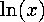<b>double log(double x)</b>

Document: The C Standard Librarydouble log10(double x)Mathematical Functions: math.hdouble exp(double x)

## double log(double x)

The return value is the natural logarithm of x, i.e.. x must be greater than zero.

McMullin@eeng.dcu.ie
Fri Mar 29 14:35:38 GMT 1996